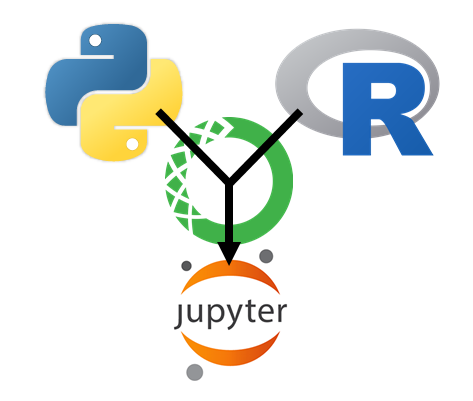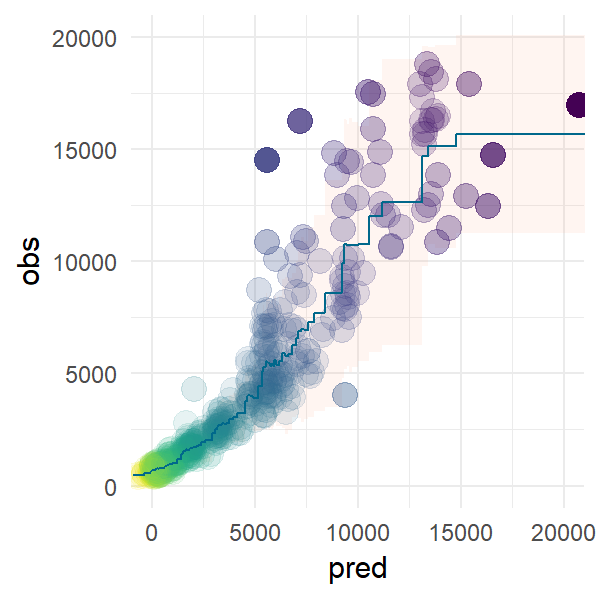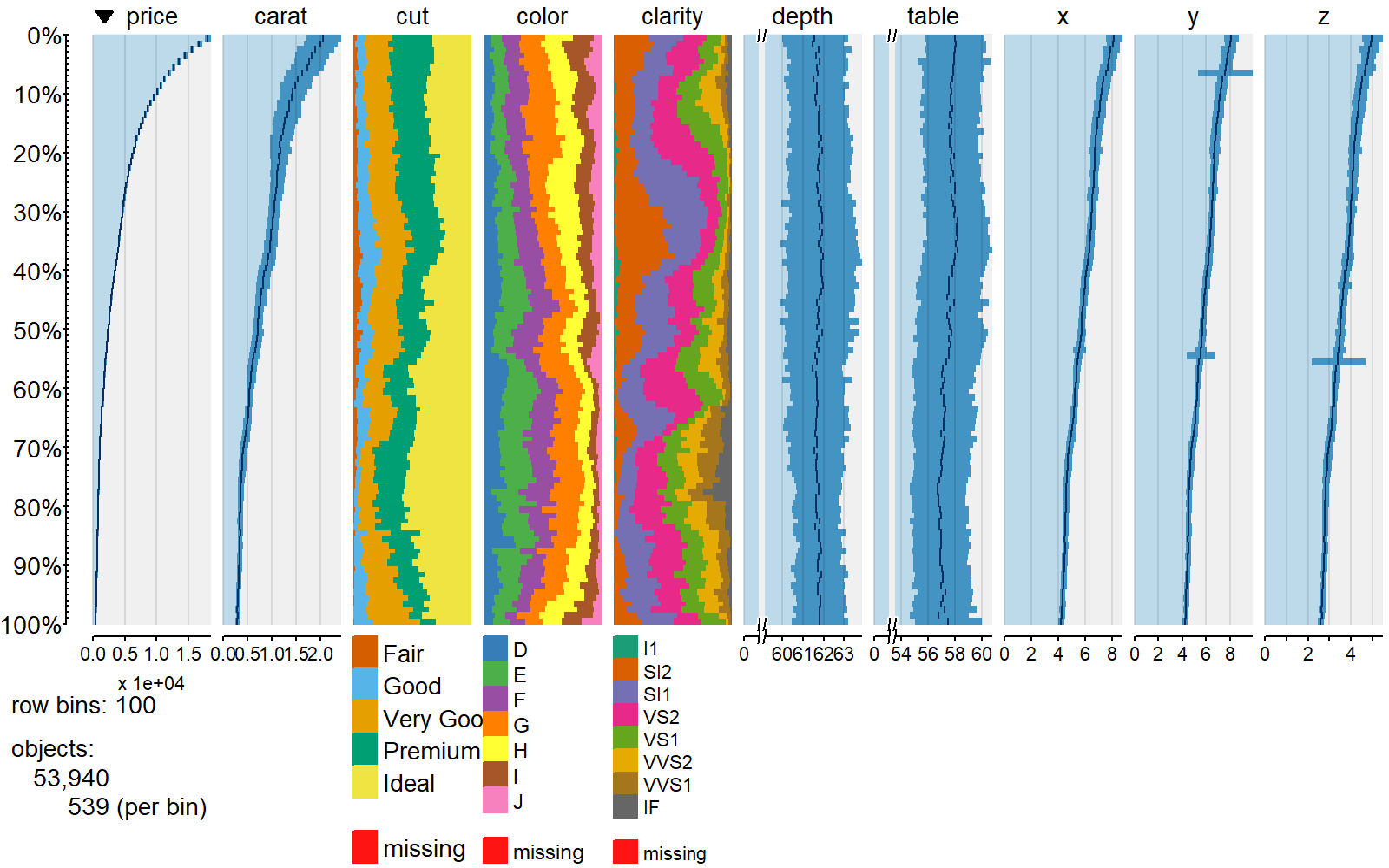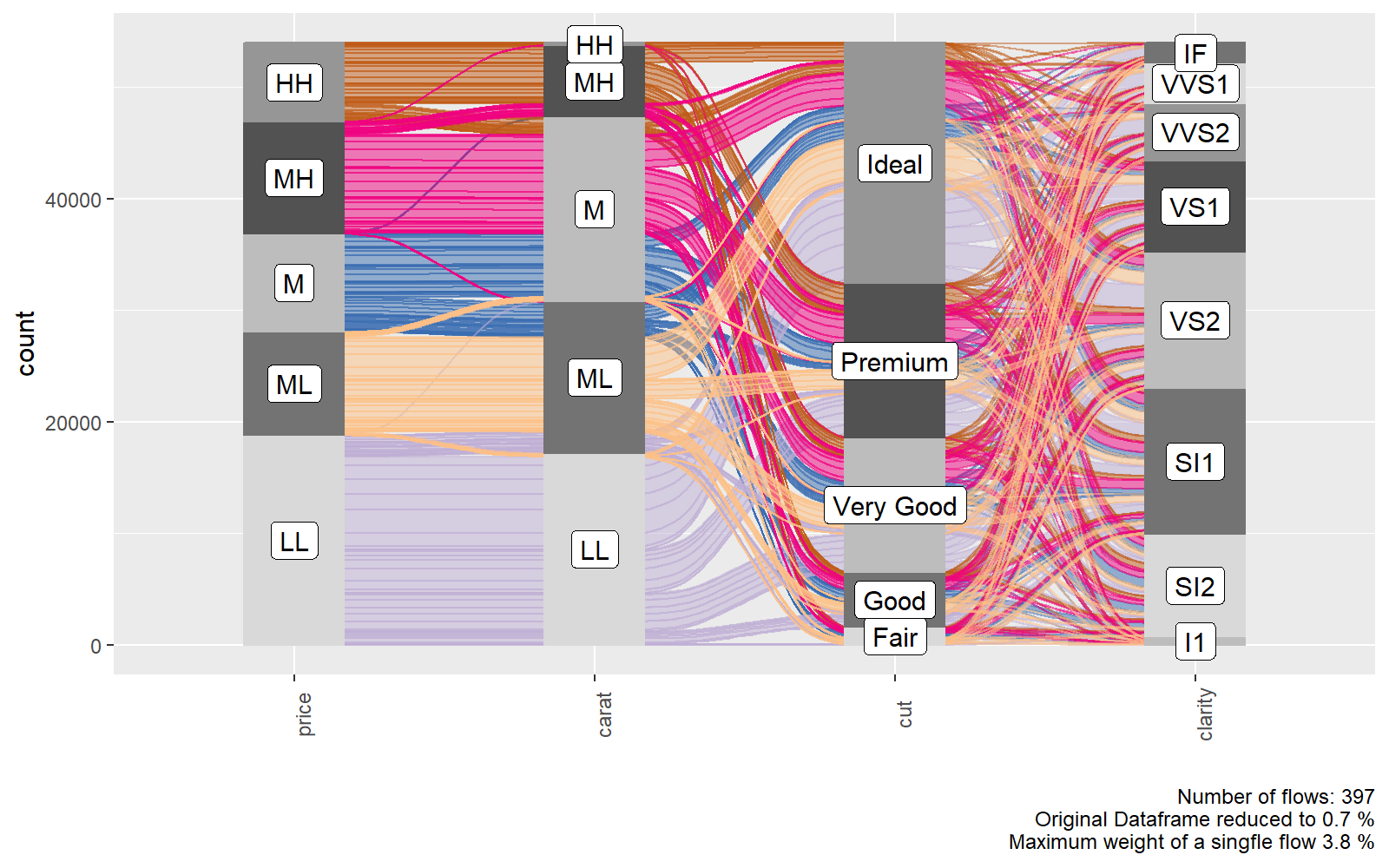Here we want to show how we can use `R` and `python` in the same jupyter notebook.

# jupyter notebooks

We first need to create a conda and install `R` and `python` and `jupyter`, then we need to activate that environment and run the `jupyter notebook` command. When creating a new notebook you will automatically use the active conda environment as a kernel.

For the sake of completeness you can also install the IRkernel and follow the instructions in order to start up a jupyter notebook using a R kernel.

When installing the package `nb_conda` you will be able to select a conda kernel from all the conda kernels on your system via the jupyter notebook GUI. For mixed environments you can select whether it should be a `R` or a `python` notebook. When you want to mix both languages inside one notebook `rpy2` will always look for the R installation circumventing jupyter. It is therefore important that you activate the appropriate mixed environment before starting jupyter.

# mixing R and python with rpy2

`rpy2` allows us to mix `R` and `python` code in our jupyter notebook by using the `rmagic` commands, which are part of the `rpy2` package.

First we import the `ipython` module from rpy2

``````%load_ext rpy2.ipython
``````
``````The rpy2.ipython extension is already loaded. To reload it, use:
``````

We can execute a line of R code using the `%R` prefix. The last returned variable is treated as cell output

``````%R X=c(1,4,5,7); sd(X); mean(X)
``````
``````array([4.25])
``````

I would recommend using `conda` which allows us to manage `python` and `R` packages in the same environment. The following code will check the `R` version jupyter is using.

``````%R d = as.data.frame( R.Version() )
%R d = d['version.string']
``````
version.string
1 R version 3.4.3 (2017-11-30)

We can get variables from the `R` instance that `jupyter` is running in the background using the `%Rget` command as cell output.

``````%Rget d
``````
version.string
1 R version 3.4.3 (2017-11-30)

We can pass variables from the `python` environment into the `R` environment using `%R -i`

``````import pandas as pd
df = pd.DataFrame( dict(a = [1,2,3], b = [4,5,6]) )

%R -i df
%R df_R = df
%Rget df_R
``````
a b
0 1 4
1 2 5
2 3 6

Similarily we can pass variables from `R` back to `python` using `%R -o`

``````%R -o df_R
df_R
print( 'type of imported R object: ' + str( type(df_R) ) )
df_R
``````
``````type of imported R object: <class 'pandas.core.frame.DataFrame'>
``````
a b
0 1 4
1 2 5
2 3 6

We can also define an entire cell as R code using `%%R` and run complex R code that uses external none-standard R packages. (Note for this code bit to run you need to create a conda environment from one of the `.yml` files found here).

``````%%R -o df

require(tidyverse)
require(oetteR)

m = lm(price ~ carat + depth, ggplot2::diamonds)

df = tibble( obs = ggplot2::diamonds\$price
, pred = predict(m, newdata = ggplot2::diamonds) ) %>%
f_prediction_intervall_raw( 'pred','obs', intervall = 0.975) %>%
f_prediction_intervall_raw( 'pred','obs', intervall = 0.025)

p = f_plot_pretty_points( dplyr::sample_n(df, 500), 'pred', 'obs' ) +
geom_ribbon(mapping = aes(ymin = pred_PI2.5_raw
, ymax = pred_PI97.5_raw
, fill = 'lightsalmon')
, data = df
, color = NA
, fill = 'lightsalmon'
, alpha = 0.1 ) +
geom_line(mapping = aes(x = pred
, y = pred_mean_raw
)
, data = df
, color = 'deepskyblue4'
#, size = 1
) +
coord_cartesian( xlim=c(0,20000), ylim = c(0,20000))

# reorganize layers
p\$layers = list(p\$layers[], p\$layers[], p\$layers[] )
``````
``````print(df)
``````
``````# A tibble: 53,940 x 6

obs  pred steps               pred_PI97.5_raw pred_mean_raw pred_PI2.5_raw

<int> <dbl> <fctr>                        <dbl>         <dbl>          <dbl>

1   337  -897 [ -897.30, -368.04)             645           492            338

2   367  -879 [ -897.30, -368.04)             645           492            338

3   367  -797 [ -897.30, -368.04)             645           492            338

4   478  -789 [ -897.30, -368.04)             645           492            338

5   386  -781 [ -897.30, -368.04)             645           492            338

6   367  -767 [ -897.30, -368.04)             645           492            338

7   425  -758 [ -897.30, -368.04)             645           492            338

8   472  -758 [ -897.30, -368.04)             645           492            338

9   367  -756 [ -897.30, -368.04)             645           492            338

10   472  -748 [ -897.30, -368.04)             645           492            338

# ... with 53,930 more rows
``````

# Plots

We can plot `R` graphics as follows using `%%R -w 5 -h 5 --units in -r 200` which sets the output to 5 x 5 inches with a resoulution of 200 dpi. Note this only works as cell magic not as in-line magic.We are using the `ggplot2` plot which we created in the previouse `R` cell.

``````%%R -w 3 -h 3 --units in -r 200
p
``````# htmlwidgets

## plotly

We can convert `ggplot2` plots to `plotly` objects and save them as `html` and embedd them in an iframe.

``````%%R
require(plotly)
pl = ggplotly(p, tooltip = c('~pred_mean_raw', '~pred_PI2.5_raw', '~pred_PI97.5_raw'))
htmlwidgets::saveWidget(pl, 'pred_intervall.html' )
``````
``````from IPython.display import IFrame

IFrame("./pred_intervall.html"
, width = 700
, height = 700)
``````

## DT

Similarly to `plotly` we can embedd dynamic datatables from the `DT` package

``````%%R

dt = oetteR::f_datatable_universal( sample_n(df, 500) )
htmlwidgets::saveWidget(dt, 'table.html' )
``````
``````IFrame("./table.html"
, width = 1100
, height = 600)
``````

# tabplot

We can use other packages from `R` to integrate other useful visualisations such as `tabplots`

``````%%R -h 5 -w 8 --units in -r 200

require(tabplot)

tableplot( select(ggplot2::diamonds, price, carat, everything()) )
``````# ggalluvial

``````%%R -h 5 -w 8 --units in -r 200

require(oetteR)

f_plot_alluvial( select(ggplot2::diamonds, price, carat, cut, clarity) )
``````# ggpubr

``````%%R -h 5 -w 8 --units in -r 200

require(ggpubr)
require(oetteR)

# generates all possible compbinations and removes pairings with P val > thresh
compare = f_plot_generate_comparison_pairs(ggplot2::diamonds, 'price', 'cut', thresh = 0.05)

ggviolin( data = ggplot2::diamonds
, x = 'cut'
, y = 'price'
, color = 'cut'
, fill = 'cut'
, palette = f_plot_col_vector74()